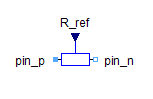Applied World Force - MapleSim Help

Applied World Force

Applies a force between ground and a frame, based on the specified input signalsConnections

 Name Description ${\mathrm{frame}}_{b}$ Force driver outboard frame $\mathrm{AFInputX}$ Real signal input specifying the applied force along the x-axis of the frame specified by the  parameter $\mathrm{AFInputY}$ Real signal input specifying the applied force along the y-axis of the frame specified by the  parameter $\mathrm{AFInputZ}$ Real signal input specifying the applied force along the z-axis of the frame specified by the  parameterParameters

 Symbol Default Units Description Modelica ID $\mathrm{Inboard}$ - Defines the frame in which the x, y and z input signals are assumed to be expressed. For the applied world force, the $\mathrm{Inboard}$ frame is the same as the $\mathrm{Inertial}$ frame. FrameSee Also